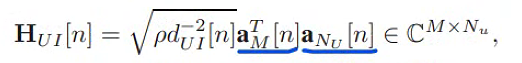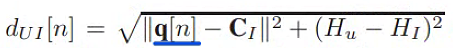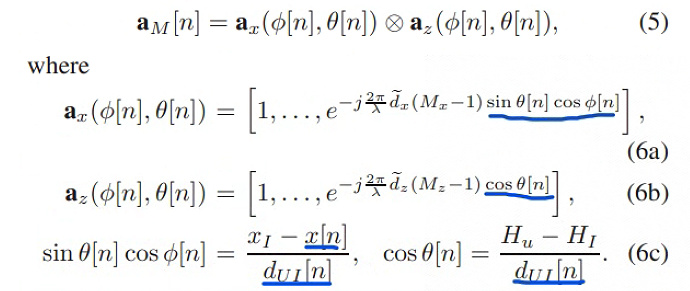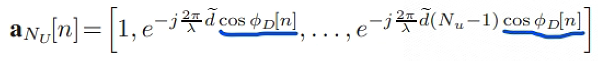# A complex channel model

``````Hello, everyone !
I have a complex channel model, do you know how to express the `HUI` by CVX ?

Expressions related to variable  are underlined in blue

variable:  q[n] = [x[n],y[n]]
``````whereIf the arguments of the trig functions are not constants (I’m assuming they are not), then the expression is neither convex nor concave,